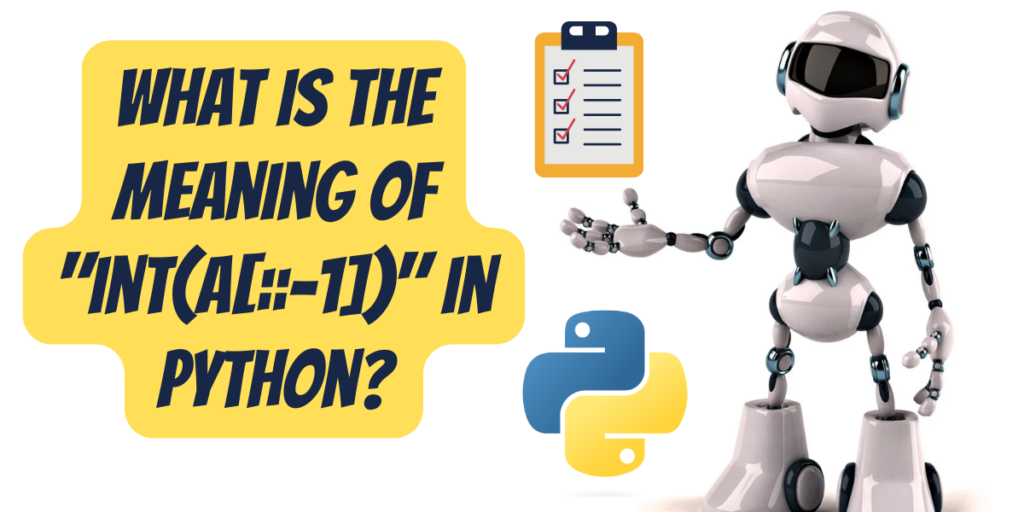# What is the meaning of “int(a[::-1])” in Python?Strings are a collection of characters. It is an immutable data type. Since python doesn’t have a character datatype specifically, it converts the elements of a string into its’ respective Unicode characters.

Syntactically, strings are declared in between double or single quotes in python. We can traverse through strings in the same way that we traverse through a list using for or while loops. Strings are also indexed in the same way that arrays or lists are indexed. The first character of a string is placed at the 0th position while the last character is placed at position N-1 where, N is the length of the string.

Strings also support a vast range of built-in functions just like lists. The `len()` function can be used to determine the length of a string. There are also other functions such as replace() and slice() which can be used for string manipulation.

## String slicing and reversing a string literal

String slicing is used when we want to remove certain characters from a string that are not important for our use. String slicing can be done from the beginning of a string or from the end of it or even from the middle. The flexibility of python functions is what makes it so popular and easy to use.

The syntax for string slicing is as follows:

```str.slice(start:stop:step)
```

or simply,

```str[start:stop:step]
```

Here, str is our string variable. The slice() function takes up three values separated by “:” .

• start: It is the first position from where the string is to be sliced. When not mentioned, it starts from the very first or 0th position.
• stop: This is the position where the string slicing is to be stopped. If the stop position has a value of -1, it means that the string is to be traversed in a reverse order. In this case, the start position if not specified, will take up the last value, that is, length of string minus 1.
• step: The increment between each slicing index. This is by default set to 1 if not explicitly mentioned in the program.

Now, sometimes we need to reverse slice a string for specific purposes. The index, -1, refers to the position before the very first element of a list, string or tuples.

Therefore, int(a[::-1]) refers to slicing a string variable named “a”, which has numbers in the form of strings in it and then converting the sliced string literal into integer literals explicitly by using the int() function. If the variable “a” has characters or alphabets, then the above statement will raise a ValueError. The a[::-1] mentions that the program has to traverse through the very end of the string “a”, up to the very first element of the string. Hence, it will give us the reverse of the string.

## Running int(a[::-1]) and analyzing the result

If you assign a value of “12345” (say) then initializing the print(int(a[::-1])) will display the reverse of the string and the data type of the variable will change from string to integer.

The int() function converts a string literal containing numbers into an integer data type. It is explicitly assigned.

Let us look at how to run the code:

```#initializing the string variable
a=input("Enter numbers as strings=") #taking user input for our string
print("The original string is=",a) #displaying the original string
print("The original datatype of the variable is=",type(a))#printing the datatype of our variable
b=(int(a[::-1]))#converting the string into an integer and reversing it
print("The reversed output is=",b)#displaying the reversed string
print("Datatype after operation=",type(b))#displaying the type after explicitly assigning it as integer.
```

The output will be:

```Enter numbers as strings=12345
The original string is= 12345
The original datatype of the variable is= <class 'str'>
The reversed output is= 54321
Datatype after operation= <class 'int'>
```

Also read: Integer to Binary String in Python.

## ValueError and how to fix it?

Now, suppose you want to reverse a string of characters using this method but when you try to convert a collection of characters into integers it will raise an inevitable ValueErrors. ValueErrors are raised when the data type of a variable is incorrectly assigned to specific functions.

If we assign this message to our “a” variable: “hi, How are you?” it will raise a value error because the int() function only accepts values with base 10.

Now, if we just remove the int() function conversion, we can easily reverse a string using this method. Let’s look at that:

```# initializing the string variable

a = input('enter string=')  # taking user input for our string
print ('The original string is=', a)  # displaying the original string
print ('The original datatype of the variable is=', type(a))  # printing the datatype of our variable
b = a[::-1]  # converting the string into an integer and reversing it
print ('The reversed output is=', b)  # displaying the reversed string
print ('Datatype after operation=', type(b))  # displaying the type after explicitly assigning it as integer.
```

The output is:

```enter string = AskPython.com
The original string is = AskPython.com
The original datatype of the variable is = <class 'str'>
The reversed output is = moc.nohtyPksA
Datatype after operation = <class 'str'>
```